# ProblemsCalculate the total binding energy of 2010 Ne.

Solution:
By Table 29.3 in the text, we see that 2010 Ne has a mass of 19.992439 u. Since Neon has 10 protons and 10 neutrons, the mass deficiency is

10 x 1.007825 + 10 x 1.008665 - 19.992439 = 0.172461 u .

This corresponds to an energy of

E = mc 2 = 0.172461 u x 931.5c 2 = 160.6 MeV .

Thus, the binding energy is 160.6 MeV, or about 8.0 MeV per nucleon.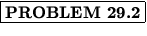Suppose you begin with 1.0 x 10- 2 g of a pure radioactive substance and 4 h later determine that 0.25 x 10- 2 g remain. What is the half-life of the substance?

Solution:
For this we will use the relation

N = N0e -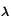t=t/T1/2,

where T1/2 = ln 2/has been used. We then have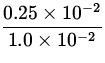= 0.25 =t/T1/2ln 0.25 =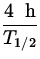ln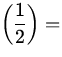-ln 2,

from which we find T1/2 = 2.0 h.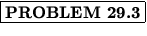The 14C content decreases after the death of a living system with a half-life of 5739 years. If the 14C content of an old piece of wood is found to be 12.5% of that of an equivalent present-day sample, how old is the piece of wood?

Solution:
This will also use the relation

N = N0e -t=t/T1/2.

With the data given, we find

0.125 =t/5739 yrln 0.125 =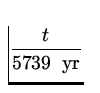ln-ln 2,

from which we deduce that t = 17,217 yr.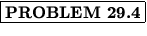Find the energy released in the alpha-decay

23892 U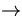23490 Th + 42 He

Solution:
For this we shall need the masses

 M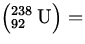238.050786 u , M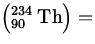234.043583 u , M4.002603 u ,
from which we find the mass difference to be

238.050786 - (234.043583 + 4.002603) = 0.0046 u .

This corresponds to an energy of

E = mc 2 = 0.0046 u x 931.5c 2 = 4.29 MeV .

Thus, the reaction releases an energy of 4.29 MeV.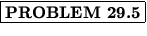Suppose that the sun consists entirely of hydrogen and that the dominant energy-releasing reaction is

4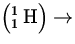42 He + 2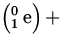2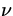+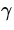.

If the total power output of the sun is assumed to remain constant at 3.9 x 1026 W, how long will it take for all of the hydrogen to be burned up? Take the mass of the sun as 1.99 x 1030 kg.

Solution:
We first find the total number of hydrogen atoms in the sun by calculating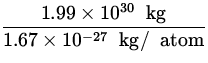= 1.192 x 1057 atoms .

Now, the reaction quoted has a mass difference of

4 x 1.007825 - (4.002603 + 2 x 0.000549) = 0.027599 u ,

which corresponds to an energy of

E = mc 2 = 0.027599 u x 931.5c 2 = 25.71 MeV .

Since each individual reaction consumes 4 Hydrogen atoms, the total energy available in the sun is

1.192 x 1057 atoms x 25.71x 106x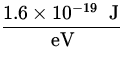= 1.225 x 1045 J .

The lifetime of the sun can then be estimated asx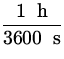x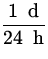x= 9.96 x 1010 yr .